Contents - Index

External_Flow_Plate_ND_local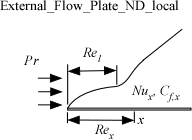Procedure External_Flow_Plate_ND_local(Re, Pr: Nusselt, C_f) returns the local Nusselt number and local friction coefficient for flow parallel to a flat plate.

Inputs:

Re - local Reynold's number [-], where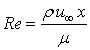where

r is the density of the fluid

m is the viscosity of the fluid

x is the position relative to the leading edge of the plate

u_infinity is the velocity of the flow

Pr - Prandtl number [-], where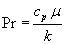where

c_p is the specific heat capacity of the fluid

k is the conductivity

Re_l - local Reynolds number [-] where transition region starts (3x103 < Re_l < 5x104)

Note that if the value of Re_l is < 0 then it is interpreted as the turbulence intensity (u`/u, as a percentage)

In this case, the empirical equation from Mayle (1991) is used.

Outputs:

Nusselt - local Nusselt number assuming a constant wall temperature[-]. The Nusselt number is defined: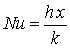where

h is the average heat transfer coefficient

C_f - local friction coefficient [-]. The friction coefficient is defined: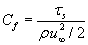where

t is the local shear stress

Notes

This procedure uses the correlations presented in Nellis and Klein (2018) to determine the local Nusselt number and friction coefficient.

The Reynolds and Prandtl numbers are based on fluid properties evaluated at the film temperature, which is the average of the free stream and the surface temperatures.

Note that the procedure External_Flow_Plate_L_ND_local provides the same functionality but uses the more accurate correlations described by Lienhard (2020) that include the impact of the transition region.

Example 1

Re=1e6

Pr=0.7

Call external_flow_plate_nd_local(Re,Pr: Nusselt,C_f)

{Solution:   Nusselt=1666 [-],   C_f=0.003752}